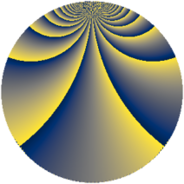# Properties

 Label 2736.3.gjLevel $2736$ Weight $3$ Character orbit 2736.gj Rep. character $\chi_{2736}(929,\cdot)$ Character field $\Q(\zeta_{18})$ Dimension $1428$ Sturm bound $1440$

# Related objects

## Defining parameters

 Level: $$N$$ $$=$$ $$2736 = 2^{4} \cdot 3^{2} \cdot 19$$ Weight: $$k$$ $$=$$ $$3$$ Character orbit: $$[\chi]$$ $$=$$ 2736.gj (of order $$18$$ and degree $$6$$) Character conductor: $$\operatorname{cond}(\chi)$$ $$=$$ $$171$$ Character field: $$\Q(\zeta_{18})$$ Sturm bound: $$1440$$

## Dimensions

The following table gives the dimensions of various subspaces of $$M_{3}(2736, [\chi])$$.

Total New Old
Modular forms 5832 1452 4380
Cusp forms 5688 1428 4260
Eisenstein series 144 24 120

## Trace form

 $$1428q + 6q^{3} - 9q^{5} + 6q^{7} + 6q^{9} + O(q^{10})$$ $$1428q + 6q^{3} - 9q^{5} + 6q^{7} + 6q^{9} + 9q^{11} - 3q^{13} + 6q^{15} + 12q^{19} - 60q^{21} + 9q^{23} - 3q^{25} + 3q^{27} - 9q^{29} - 3q^{31} - 93q^{33} + 225q^{35} - 24q^{37} + 12q^{39} + 99q^{41} - 285q^{43} - 3q^{45} + 9q^{47} + 9486q^{49} + 6q^{51} - 63q^{55} - 6q^{57} + 9q^{59} - 3q^{61} - 132q^{63} - 9q^{65} + 3q^{67} - 3q^{69} - 84q^{73} - 324q^{75} - 18q^{77} + 3q^{79} - 90q^{81} - 3q^{85} + 3q^{87} + 735q^{91} + 21q^{93} + 9q^{95} - 183q^{97} + 249q^{99} + O(q^{100})$$

## Decomposition of $$S_{3}^{\mathrm{new}}(2736, [\chi])$$ into newform subspaces

The newforms in this space have not yet been added to the LMFDB.

## Decomposition of $$S_{3}^{\mathrm{old}}(2736, [\chi])$$ into lower level spaces

$$S_{3}^{\mathrm{old}}(2736, [\chi]) \cong$$ $$S_{3}^{\mathrm{new}}(171, [\chi])$$$$^{\oplus 5}$$$$\oplus$$$$S_{3}^{\mathrm{new}}(342, [\chi])$$$$^{\oplus 4}$$$$\oplus$$$$S_{3}^{\mathrm{new}}(684, [\chi])$$$$^{\oplus 3}$$$$\oplus$$$$S_{3}^{\mathrm{new}}(1368, [\chi])$$$$^{\oplus 2}$$